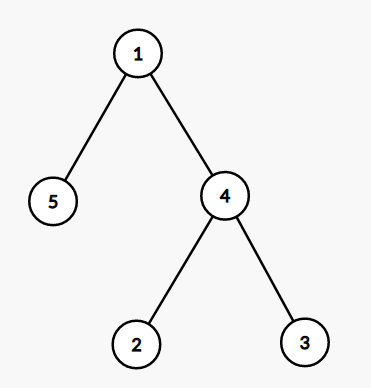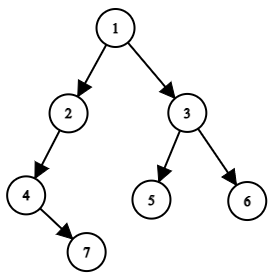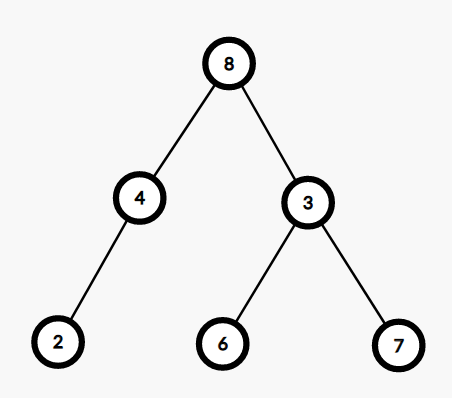Problem title
Difficulty
Avg time to solve

Minimum XOR
Easy
--
Minimum Maximum Value
Hard
--
Most Frequent Prefix
Moderate
--
Count Substrings With K Ones
Moderate
--
Minimum Removals
Moderate
20 mins
Milk flow
Moderate
15 mins
The Maze ll
Hard
25 mins
Alice And The Game Of Coins
Moderate
--
Ninja And The Pizzas
Easy
--
Count Subsequences With Given Form
Moderate
--

# Pairs Violating BST

Difficulty: MEDIUM
Avg. time to solve
25 min
Success Rate
75%

Problem Statement

#### BST tree has the following properties:-

``````1. For every node, it is greater than or equal to its left child and less than or equal to its right child.
2. Node is greater than equal to the maximum value of its left subtree and less than the minimum value of its right subtree.
3. The maximum in the left sub-tree must be less than equal to the minimum in the right subtree.
``````
##### For example in the following tree:-``````The numbers of pairs violating BST property are:-

(1,5) : 1 should be greater than its left child value.

(4,3) : 4 should be less than its right child value.

(5,4),(5,3):  Maximum of left subtree of 1 is 5 greater
than 2 and 3 of its right subtree.
``````
##### Input Format:
``````The first line contains an integer 'T' which denotes the number of test cases. Then the test cases are as follows.

The first line of each test case contains elements of the tree in the level order form. The line consists of values of nodes separated by a single space. In case a node is null, we take -1 in its place.
````````````For example, the input for the tree depicted in the above image would be :

1
2 3
4 -1 5 6
-1 7 -1 -1 -1 -1
-1 -1
``````

#### Explanation :

``````Level 1 :
The root node of the tree is 1

Level 2 :
Left child of 1 = 2
Right child of 1 = 3

Level 3 :
Left child of 2 = 4
Right child of 2 = null (-1)
Left child of 3 = 5
Right child of 3 = 6

Level 4 :
Left child of 4 = null (-1)
Right child of 4 = 7
Left child of 5 = null (-1)
Right child of 5 = null (-1)
Left child of 6 = null (-1)
Right child of 6 = null (-1)

Level 5 :
Left child of 7 = null (-1)
Right child of 7 = null (-1)
``````
##### Note :
``````The above format was just to provide clarity on how the input is formed for a given tree.

The sequence will be put together in a single line separated by a single space. Hence, for the above-depicted tree, the input will be given as:

1 2 3 4 -1 5 6 -1 7 -1 -1 -1 -1 -1 -1
``````

#### Output format :

``````For each test case, print a single line containing a single integer denoting the number of pairs violating the BST property.

The output of each test case will be printed in a separate line.
``````
##### Note:
``````You do not need to print anything, it has already been taken care of. Just implement the given function.
``````
##### Constraints
``````1 <= 'T' <= 10
1 <= Number of nodes in Tree <= 5000
1 <= Value of nodes <= 10 ^ 5

Where 'T' is the number of test cases.

Time Limit: 1 sec.
``````
##### Sample Input 1:
``````1
8 4 3 2 -1 6 7 -1 -1 -1 -1 -1 -1
``````
##### Sample Output 1:
``````5
``````
##### Sample Output Explanation
`````` For the above input tree is:-
````````````Thus pairs violating BST property are:-
(8,3) :- Greater than its right child.
(8,6) :- Greater than node on its right side.
(8,7) :- Greater than node on its right side.
(3,6):- The value of 3 is not more than its left child.
(4,3):- The value in the left subtree is greater than the
value of the right subtree.

Thus the number of pairs is 5.
``````
##### Sample Input 2:
``````2
35 44 26 -1 50 37 20 -1 -1 43 36 -1 -1 -1 -1 -1 -1
49 48 26 32 36 40 31 30 -1 25 40 -1 -1 -1 40 -1 -1 -1 -1 -1 -1 -1 -1
``````
##### Sample Output 2:
``````24
25
``````Console